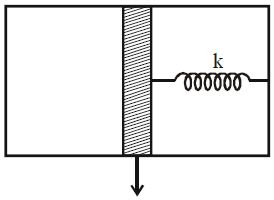# Heat Capacity + Vaccum + Springs +Heat!The figure shows a system with adiabatic walls, a piston and a spring. The space on the left side of the piston is filled with 1 mole of an ideal mono atomic gas and right side is a vacuum with a spring. Initially the system is in equilibrium. Now the gas is heated. Molar heat capacity of the gas in this case is $C=\frac{nR}{2}$ . What is the value of $n$ is?

(Neglect heat capacities of wall, piston & spring)

×